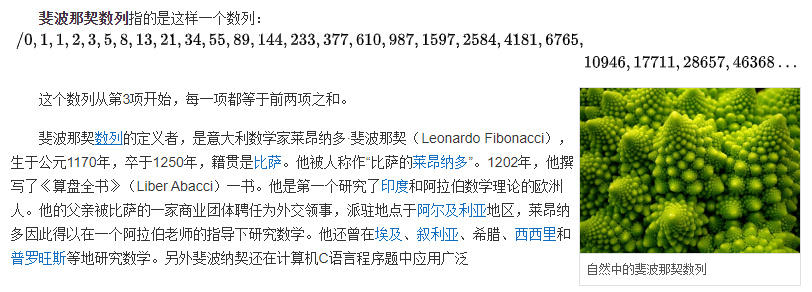• 建站日期：2019-12-01
• 文章总数：1378 篇
• 评论总数：1517 条
• 分类总数：20 个
• 最后更新：9月24日

# [JAVA基础算法] 斐波那契数列### 首先，先说一下什么是 斐波那契数？### 递推公式

``Fn=F(n-1)+F(n-2)（n>=2，n∈N*）``

### 题目：

``````1,1,2,3,5,8,13,21,... 称为斐波那契数列

### 代码：

``````public class Main {
//递归方法
public static long f(int n) {
if(n == 1 || n == 2) {  //斐波那契数第一位和第二位都是1
return 1;           //所以无论到第一位还是第二位直接返回1即可
}
return f(n-1)+f(n-2) ;
}
//for循环方法
public static long xfor (int n) {
long a1 = 1, a2 = 1;      //给出斐波那契数第一位和第二位
for(int i = 3; i <= n; i++) {
long a3 = a1;
a1 = a2;
a2 = a3 + a1;   //后一项等于前两项之和
}
return a2;
}

public static void main(String[] args) {
int n = 20;
System.out.println(xfor(n));
System.out.println(f(n));
}

}``````

-- 展开阅读全文 --

« 上一篇
CPU-Z v1.93.0 中文单文件版

### 发表评论HI ! 请登录